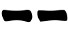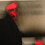## Saturday 21 May 2016

Four coins can be used to easily generate hexagram lines with yarrow stalks probabilities.

This method has been devised by Stuart Anderson and proceeds as follows:

1. Pick three identical coins and one coin that is notably different from the others
2. Throw the four coins;
3. Look at the single different coin:
• If it's tail, draw;
• If it's head, draw;
4. Look at the four coins, if among them there are exactly three head, it is a moving line.
5. Repeat steps 1-4 drawing hexagram lines from bottom to top.

#### Probabilities

The table below summarizes the possible outcomes of this metods, the results that generate a moving line are highlighted in red.

By counting, it's easy to see that the probabilities are:
Prob(6) = 1/16
Prob(8) = 7/16
Prob(7) = 5/16
Prob(9) = 3/16
Prob(yin) = Prob(yang) = 1/2

1.2.